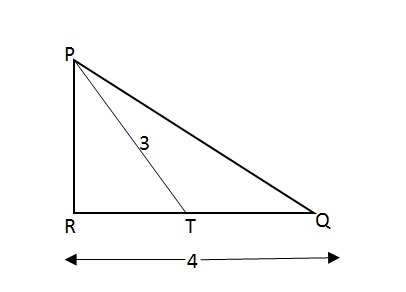# CAT Quantitative Aptitude Questions | Geometry Questions for CAT - Triangles

###### CAT Questions | CAT Geometry Questions | Triangles

The question is from the topic triangles. It is about a right angle triangle and a point on the adjacent side which divides the triangle into two. CAT Geometry questions are heavily tested in CAT exam. Make sure you master Geometry problems. We have to find the possible ratio of two sides.

Question 41: A right angled triangle PQR is such that ∠PRQ = 90° and QR = 4 cm T is a point on QR such that PT = 3 cm, and perimeter of triangle PQT = Perimeter of triangle PTR Then, QT/TR takes the value:

1. QT/TR < 1/3
2. 1/3 < QT/TR < 1
3. QT/TR > 1
4. can’t be determined

## Best CAT Coaching in Chennai

#### CAT Coaching in Chennai - CAT 2021Online Batches Available Now!

##### Method of solving this CAT Question from Triangles: Knowing the properties of right angle triangle would help.Given P (∆PRT) = P (∆PTQ)
=> PR + RT + PT = PT + TQ + PQ
=> PR + RT = QT + PQ
Since, PQ > PR (PQ is hypotenuse)
=> RT > QT
=> QT/TR < 1
Also, given PT = 3 so RT < 3 (PT is hypotenuse)
Since, RT + TQ = 4, when RT = 3, TQ = 1
But Since RT < 3
∴ QT/TR > 1/3

The question is "QT/TR takes the value"

##### Hence, the answer is 1/3 < QT/TR < 1.

Choice B is the correct answer.

###### CAT Coaching in ChennaiCAT 2021Enroll at 49,000/- 44,000/-

Online Classroom Batches Starting Now!

###### Best CAT Coaching in ChennaiPrices slashed by Rs 4000/-

Attend a Demo Class

## CAT Preparation Online | CAT Geometry questions Videos On YouTube

#### Other useful sources for Geometry Question | Geometry Triangles Circles Quadrilaterals Sample Questions

##### Where is 2IIM located?

2IIM Online CAT Coaching
A Fermat Education Initiative,
58/16, Indira Gandhi Street,
Kaveri Rangan Nagar, Saligramam, Chennai 600 093

##### How to reach 2IIM?

Phone: (91) 44 4505 8484
Mobile: (91) 99626 48484
WhatsApp: WhatsApp Now
Email: prep@2iim.com